## computer programsJOURNAL OFSYNCHROTRONRADIATION
ISSN: 1600-5775
Volume 13| Part 6| November 2006| Pages 489-493

## Calibration of spectra from dispersive XAS beamlines

aDepartment of Physics, University of Warwick, Coventry CV4 7AL, UK
*Correspondence e-mail: m.p.ruffoni@warwick.ac.uk

(Received 26 April 2006; accepted 25 September 2006)

The DXAS Calibration computer program provides a quantitative and automated solution to the problem of calibrating spectra from dispersive XAS beamlines. Such spectra, obtained in arbitrary energy units, are calibrated with respect to the absorption features of a supplied reference spectrum, which has been obtained under similar conditions on a calibrated beamline. In addition to basic energy coordinate transformation parameters, DXAS Calibration supplies instrument corrections to compensate for mismatches in instrument response functions between the dispersive and reference beamlines.

### 1. Introduction

Dispersive XAS beamlines, such as ID24 of the ESRF, utilize a single Si(111) Bragg diffracting or Si(311) Laue diffracting polychromator crystal which is elliptically bent and illuminated by white X-rays, typically over an area of 10 µm vertically by 40 mm horizontally, to produce a wavelength-dispersive diffracted beam (ESRF, 2001).

This beam, spread out into a broad horizontal fan, converges to a focus, allowing a sample to be simultaneously illuminated by X-rays of wavelengths corresponding to an energy range of typically 700–1500 eV. Beyond this focal spot, each wavelength component diverges again, and is detected by a position-sensitive CCD array of 18 pixels × 1152 pixels in the case of ID24, as shown in Fig. 1.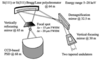Figure 1 The optics of beamline ID24 of the ESRF (ESRF, 2005).

As a result of this geometry, the energies of X-rays detected in each pixel of the CCD (which are related to X-ray wavelength given E = hc/λ) are dependent on the source and optics settings, on the amount of bending in the polychromator crystal, and on the distance between the detector and the focal spot, and as such cannot be accurately determined prior to measurement. It is therefore required that calibration be carried out a posteriori with respect to a spectrum of known calibration.

Thus, each absorption spectrum is acquired on a relative scale and presented to the user as a function of CCD channel (pixel) number. The user is then required to manually calibrate the energy and absorption scales, converting from CCD channel number to a real energy scale and from relative to an absolute absorption scale. This is usually accomplished by comparing an acquired spectrum with a reference obtained from a standard calibrated XAS beamline such as BM29, the X-ray absorption spectroscopy beamline at the ESRF. Some tools already exist to aid the user in this task (ESRF, 2001), but none are fully automated and therefore accuracy is limited by human judgement.1 Additionally, few are dedicated to the calibration requirements of dispersive-XAS beamlines and hence can often be difficult to use. DXAS Calibration solves these problems by providing an automated graphically driven calibration solution that is dedicated to the problems presented by dispersive-XAS beamlines.

### 2. The calibration process

#### 2.1. Calibration parameters

Assuming that the dispersive and reference spectra were acquired under the same environmental conditions, from the same sample material and also, for now, that the instrument response functions of the dispersive and reference beamlines were identical, then calibration is reduced to calculating the values of a set of parameters necessary to transform the channel number `pseudo-energy' axis to an absolute energy scale, and relative absorption to absolute absorption. Algorithmically, these parameters are the coefficients of two polynomials: one for energy calibration and one for absorption normalization, so that, for each pixel i,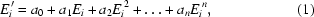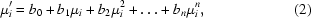where Ei and μi are the original energy (which may be just the pixel number) and absorption coefficients, respectively, and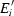and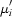are the transformed coordinates. As a result, a0 and b0 perform a simple translation of the spectrum, a1 and b1 stretch the spectrum in a linear fashion, and higher-order coefficients induce non-linear deformations in the spectrum. Setting a1 and b1 to 1 and all other parameters to 0 will return the original spectrum as it was experimentally recorded. The order n of the polynomial is specified by the user, and is typically between 2 and 4. An option also exists allowing a user to correct any absorption drift if necessary. Given that all parameters ai and bi are independent, they may be inserted into a single vector a for use by the calibration algorithm.

Frequently, these parameters alone will suffice in generating an accurate calibration; however, it is also important to consider the case where our final assumption fails, and the instrument functions of the dispersive and reference beamlines differ. When this happens, additional instrument compensation parameters are required, which are discussed in more detail in §3.

#### 2.2. Calibration algorithm

The DXAS Calibration code implements a Levenberg–Marquardt algorithm for general non-linear least-squares fitting of an arbitrary number of parameters (Adby & Dempster, 1974; Press et al., 1992). For the purpose of creating a calibration algorithm within the assumptions given above, we must state a priori that it is possible to select some set of parameters, a, which when applied to a dispersive spectrum, f(E, a), should in theory reduce any differences in absorption structure between it and the reference spectrum, fref(E), to a minimum. We therefore define some least-squares cost function that measures the differences in structure for any potential solution, and work to minimize it. Given also that the code is always supplied with sets of spectral data, discretely sampled at energies Ei, the differences may simply be evaluated at each data point as yi(a) = f(Ei, a) − fref(Ei).

#### 3.1. Matching beamline instrument functions

In some cases, especially at higher X-ray energies or with thick specimens where the absorption thickness product is greater than e.g. 1.5, the instrument functions of dispersive beamlines differ significantly from those of standard XAS beamlines. In these cases, the stated calibration condition that the cost function can be reduced to an acceptable minimum, simply by applying translations and deformations to the uncalibrated spectrum of the polynomial form (1)and (2), is no longer valid. It is therefore necessary to match the instrument functions of the dispersive and reference beamlines so that, idealistically, two spectra obtained from the same sample, under the same environmental conditions, are identical.

This can be achieved by convolving the reference transmission spectrum (which is of a higher resolution than the same spectrum obtained from a dispersive beamline) with instrumental weight functions that compensate for the differences in X-ray source and optics between the two beamlines, the optimal characteristics of which can again be obtained from a Levenberg–Marquardt algorithm.

Klug & Alexander (1974) describe several types of instrument corrections that are applicable to powder diffractometry, but which are adaptable to our situation, and could be applied to the reference spectrum in order to match its instrument function to that of the dispersive beamline. However, in the specific case of dispersive spectra from ID24 and reference spectra from a standard XAS beamline such as BM29, we empirically find only two such functions to be required.

The first is a normalized Lorentzian of the form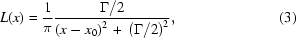where Γ is found to be 2.35 eV at the Sr K edge (16.105 keV). This function is applied to the transmission data (i.e. the data prior to taking the log to convert to absorption) and compensates for the so-called thickness effect where differences in the tails of the instrument functions between the reference monochromator and dispersive polychromator, as a function of angular deviation from the diffracting Bragg angle, induce differences in the absorption spectra. The Lorentzian form of the tails is predicted from perfect crystal theory. These Lorentzian tails to the diffracted beam reduce its monochromacity, and hence are clearly undesirable. As a consequence, XAS beamlines commonly utilize a two-crystal Si(111) or Si(311) monochromator, arranged in the parallel configuration, so that the twice diffracted beam suppresses these tails. Dispersive beamlines, however, implement only a single Si(111) or Si(311) crystal to select X-ray wavelengths. As a result, the tails on the angular reflectivity profile are unattenuated, distorting the fine structure. For calibration purposes, convolving the reference transmission spectrum with the Lorentzian (3) will reintroduce the broadening effects eliminated by the two-crystal monochromator, matching its characteristic reflectivity profile to that of the dispersive spectrum. The second instrument weighting function is asymmetric and is a normalized exponential of the form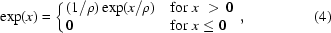where ρ is found to be 1.25 eV at the Sr K edge. This term arises due to differences in X-ray penetration into the monochromator crystals on the two beamlines. For a perfect crystal of Si(111), diffracting in the dynamical regime, X-ray penetration into the crystal is calculated from the extinction length to be of the order of 1 µm. However, a dispersive beamline has a bent crystal polychromator, which in turn has bent lattice planes that break the conditions necessary for purely dynamical diffraction. Equally, the crystal is not deformed sufficiently for purely kinematical diffraction to occur. Therefore, the X-ray penetration depth lies somewhere between the extinction and absorption lengths, the values predicted for each regime, respectively. This penetration can be increased further in the presence of damage to the surface of the crystal that may have resulted from its preparation process.

The result is that, on the dispersive beamline, X-rays of a given energy diffract from many more lattice planes of greater depth, which in turn spatially smears the diffracted beam perpendicular to the planes. These X-rays are therefore detected in several pixels along the length of the CCD, broadening the spectrum. Given also that the beam intensity decays exponentially as it penetrates the crystal, with a sharp discontinuity at the crystal surface, the smeared intensity of a single wavelength component of the diffracted radiation will decay exponentially in space as shown in equation (4). This introduces an erroneous asymmetrical energy shift in the observed structure. On ID24, the geometry dictates that this shift is towards higher energies. The observed penetration depth as a function of the radius of curvature of the polychromator, p(R), may be calculated from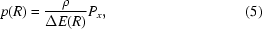where ΔE(R) is the CCD energy resolution and Px is the horizontal size of each CCD pixel. On ID24, Px = 25.0 µm, and, at the Sr K edge, ΔE(R) = 1.26 eV, giving p(R) = 24.6 µm.

Other effects, such as from samples themselves, may also degrade dispersive measurements with respect to those from reference beamlines. One such example is a reduction in resolution and white-line intensity owing to small-angle scatter by BN-based powder samples (Hagelstein et al., 1998). However, these effects are neglected here for two reasons. Firstly, they generally represent only a small change in the XAFS spectrum as a whole, and are found not to affect the overall quality of calibration fits; and, secondly, such effects may be avoided by selecting suitable samples for calibration measurements; for instance, by making such measurements through reference foils rather than powder specimens.

#### 3.2. Implementation of convolutions

Convolution theorem dictates that the convolution of two functions, e.g. f and g, is given by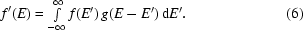This may be evaluated by taking the Fourier transforms of f and g, multiplying them together, and back-transforming the resulting function. Therefore, computational problems involving convolutions are commonly tackled using a fast Fourier transform code. The result is very rapid evaluation of the convolution, which is ideal for iterative applications as implemented here, but comes at the expense of potentially introducing transform artefacts to the spectrum.

It is also possible, however, to take the discretely sampled spectrum data, f, and perform the convolution numerically at each data point as long as the function with which it is being convolved, g, goes to zero as E′ goes to infinity. Fortunately, this is the case with equations (3)and (4). Evaluating the convolution this way will guarantee the absence of any Fourier transform artefacts, but at the expense of speed. Given the importance of an accurately calibrated spectrum, DXAS Calibration evaluates any required convolutions using this latter numerical technique.

The speed penalty resulting from this choice of algorithm is not too severe in practice. Given that a typical sampling interval for an XAS beamline is between 0.1 and 1.0 eV, and that the characteristic widths of both the Lorentzian and exponential convolution functions are of the order of a few eV, the convolution may be accurately evaluated by summing contributions from only a few tens of data points. As a result, evaluating the convolution, and Marquardt difference and derivative matrices of a spectrum spanning 1000 eV (sampled every 0.5 eV) with a Lorentzian of Γ = 3.40 eV and an exponential of ρ = 1.25 eV, takes approximately 2 s on a Pentium IV 3.0 GHz processor.

#### 3.3. Instrument parameter integration

Without instrument function compensation, the output parameters from the coordinate transformation algorithm may be considered optimal after just one execution. However, if the instrument functions between dispersive and reference beamlines differ enough to require additional instrument function calibration, the optimal parameters for coordinate transformation are dependent on the convolution functions that have been applied to the reference spectrum. The instrument parameters must therefore be optimized first. Unfortunately the reverse is also true. In order to optimize the instrument parameters on the reference spectrum, a dispersive spectrum with calibrated axes is required. This circular argument means that neither the coordinate transforms nor instrument corrections may be optimized with just one execution of their respective fitting algorithms. The solution must therefore be obtained by executing each algorithm in turn, allowing it to improve its solution with respect to the other, and iterating until self-consistency is reached; that is, when neither algorithm is capable of reducing the differences in absorption structure any further.

### 4. Calibration of XAS spectra from Fe and SrF2

#### 4.1. Calibration at the Fe K edge

DXAS Calibration has been successfully tested on data obtained from ID24 at the Fe K edge (7.112 keV) of a polycrystalline Fe foil. At this energy the instrument function mismatch between ID24 and BM29, the chosen reference beamline, was minimal, and therefore calibration could be achieved without the implementation of any instrument corrections. The fit parameters are therefore only represented by coordinate transformations and background corrections. A cubic polynomial (four parameters) was fitted to the energy axis, and a linear polynomial (two parameters) was fitted to the absorption axis. Background correction was performed by fitting a six-coefficient Chebyshev polynomial to the residual differences between the two spectra and subtracting it.

The reference spectrum used in this calibration was obtained from a similar Fe foil, but from one of a thickness such that the jump at the edge, Δμx, was approximately 1.0.

An initial estimate of the parameter values was passed to the calibration algorithm, along with an initial Marquardt scale factor of 1.0 × 10−3. Calibration then optimized the parameters, aborting after seven failed attempts to reduce the spectral differences, and resulted in a solution with residual differences at each point of less than 0.4% of the average absorption. These final parameter values, and also the initial estimate values, are given in Table 1. The calibration solution is shown in Fig. 2.

 Table 1Estimated and optimized parameter values for calibration at the Fe K edge
Parameter Estimate Calibrated value
a0 7.027 × 103 7.032 × 103
a1 3.038 × 10−1 2.951 × 10−1
a2 0 −7.356 × 10−6
a3 0 1.178 × 10−8
b0 7.929 × 10−1 7.185 × 10−1
b1 5.854 × 10−1 9.324 × 10−1
Background Parameter value
x0 9.120 × 10−2
x1 6.384 × 10−2
x2 1.599 × 10−2
x3 −6.710 × 10−3
x4 6.368 × 10−3
x5 8.137 × 10−3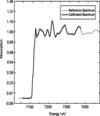Figure 2 Calibration of an ID24 Fe K-edge spectrum with respect to a similar one obtained from BM29. Both spectra were obtained from samples of polycrystalline iron foil. At the Fe K edge, the mismatch in instrument functions between ID24 and BM29 is negligible, and so this calibration consists only of polynomial-based coordinate transformations and a background correction.

#### 4.2. Calibration at the Sr K edge

At the Sr K edge (16.105 keV), best results are obtained from a Laue diffracting crystal, since many distortions become present at such high energies in Bragg geometry (Hagelstein et al., 1995). However, for the purpose of testing this code, measurements were made using Bragg geometry, where the instrument functions of ID24 and BM29 differ significantly. As such, successful energy-scale calibration required use of instrument correction parameters. Both the Lorentzian correction for diffraction monochromacity, and exponential correction for polychromator transparency, were utilized. Polynomial coordinate transformations again consisted of a cubic polynomial for energy calibration, and a linear polynomial for absorption normalization. Background correction again consisted of a six-coefficient Chebyshev polynomial. The BM29 reference spectrum was obtained from a similar SrF2 pellet as that used on ID24, which again had an edge jump of approximately 1.0.

The initial estimate and calibrated parameter values are shown in Table 2, with the calibrated solution shown in Fig. 3. The BM29 reference spectrum is shown in Fig. 4before and after the application of the instrument correction functions. The overall product of the two correction functions is shown as an insert. Some systematic differences between the ID24 and BM29 spectra persist after calibration, which most likely originate from inhomogeneities in the powdered pellets. Despite these, residual spectral differences at each point are approximately 0.7% of the average absorption, with fine-structure oscillations in calibrated and reference spectra coincident.

 Table 2Estimated and optimized parameter values for calibration at the Sr K edge
Parameter Estimate Calibrated value
a0 1.571 × 104 1.569 × 104
a1 1.228 × 100 1.220 × 100
a2 0 3.147 × 10−4
a3 0 −4.3507 × 10−7
b0 6.190 × 10−1 7.185 × 10−1
b1 9.205 × 10−1 9.324 × 10−1
Γ 1.00 eV 2.35 eV
σ 1.00 eV 1.25 eV
Background Parameter value
x0 −1.092 × 10−2
x1 1.231 × 10−1
x2 5.224 × 10−2
x3 −4.072 × 10−3
x4 −1.915 × 10−3
x5 8.419 × 10−3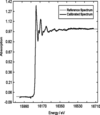Figure 3 Calibration of an ID24 Sr K-edge spectrum with respect to a similar one obtained from BM29. Both spectra were obtained from BN-based pellets of powdered SrF2. At the Sr K edge, there is significant deviation in instrument functions between ID24 and BM29, so, in addition to coordinate calibration and background correction, convolution-based instrument corrections have also been applied to the BM29 reference spectrum in order that its instrument function matches that of ID24.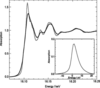Figure 4 The Sr K edge acquired from BM29, shown before (grey) and after (black) the application of instrument corrections necessary to match its overall instrument function to that of ID24. The product of the two correction functions, Lorentzian and exponential, is shown as an insert.

### 5. Conclusions

DXAS Calibration provides a quantitative and automated approach to the problem of calibration of dispersive XAS spectra. While providing a solution to the primary problem of coordinate transformation, it also moves beyond current techniques to consider calibration of spectra against references obtained from beamlines with significantly different instrument response functions. This not only allows for calibration of spectra within a more general framework, but also provides quantitative information on how dispersive beamlines perform with respect to others.

The core of DXAS Calibration is coded in cross-platform compatible C++ with a Visual Basic graphical user interface for Windows users. The source code, a windows executable file, the examples given here and comprehensive instructions are included in the package. All components of the code are freeware, and are released under the conditions of the GNU General Public License.

### Footnotes

1Subsequent to submission, the referee has pointed out that a calibration program exists in the commercial WinXAS software (http://www.winxas.de ), but this seems to be semi-automatic.

### Acknowledgements

The authors would like to thank the beamline staff of ID24 and BM29, particularly S. Pascarelli, O. Mathon and A. Trapananti, for their ongoing help and support for this project.

### ReferencesJOURNAL OFSYNCHROTRONRADIATION